# How to

17 Oct 201812 minutes to read

## How to set autocomplete default value

You can set a value into autocomplete at initial rendering using `Value` property. It is used to select a single value from the autocomplete widget at initial rendering state.

Refer to the following code.

``<ej:Autocomplete ID="selectState" runat="server" DataTextField="countryName" DataUniqueKeyField="index" Value="Arizona" />``
``````namespace SyncfusionASPNETApplication1
{
public partial class _Default : Page
{
protected void Page_Load(object sender, EventArgs e)
{
this.selectState.DataSource = new LocalData().GetDataItems().ToList();
}
}
public class LocalData    {
public LocalData(string _index, string _countryName) {
this.index = _index;
this.countryName = _countryName;
}
public LocalData() { }
public string index { get; set; }
public string countryName { get; set; }
public List<LocalData> GetDataItems()
{
List<LocalData> data = new List<LocalData>();
return data;
}
}
}``````

The following output is displayed as a result of the previous code example.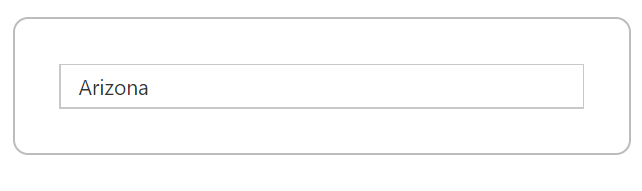If you set autocomplete `Value` property as a string that is present in the data source, the hidden input value holds the corresponding `Key` value. This is used for validation purpose in the autocomplete. For example, ‘Arizona’ text holds the key value of ‘s3’. The autocomplete default value ‘Arizona’ is present in the ‘countryName’ of data source and also this field is mapped for autocomplete `Text` property. So, hidden input value holds the ‘s3’ value.

The following screenshot illustrates the previous hidden input state of code.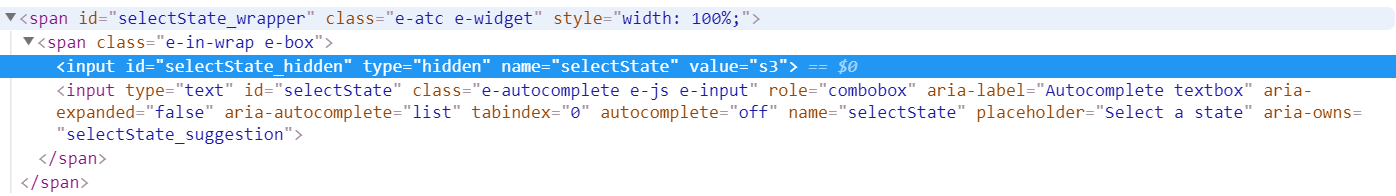If you set autocomplete `Value` property as a string that is not present in the data source, the hidden input value holds the autocomplete value. For example, if autocomplete default value is ‘New York’ and not present in the ‘countryName’ of data source, the hidden input value holds the ‘New York’string.

Refer to the following code sample.

• RAZOR
• ``<ej:Autocomplete ID="selectState" runat="server" DataTextField="countryName" DataUniqueKeyField="index" Value="New York" />``

The following screenshot illustrates the previous hidden input state of code.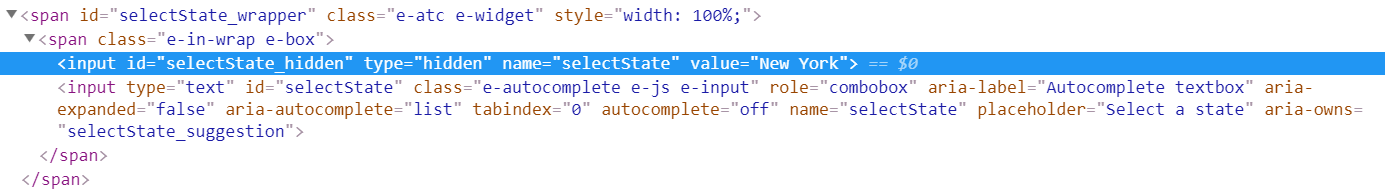### Remote data

The remote data also allows you to set the default value into autocomplete using `Value` property.

Refer to the following code sample.

``````<ej:Autocomplete ID="selectState" runat="server" DataTextField="countryName" DataUniqueKeyField="index" Query="ej.Query().requiresCount()" Value="Two">
</ej:Autocomplete>``````
``````namespace SyncfusionASPNETApplication1
{
public partial class _Default : Page
{
protected void Page_Load(object sender, EventArgs e)
{
}
[WebMethod]
[ScriptMethod(ResponseFormat = ResponseFormat.Json)]
public static object Data(Syncfusion.JavaScript.DataManager value)
{
var list = new List<AutocompleteModel>();
list.Add(new AutocompleteModel() { index = "1", countryName = "One" });
list.Add(new AutocompleteModel() { index = "2", countryName = "Two" });
list.Add(new AutocompleteModel() { index = "3", countryName = "Three" });
IEnumerable Data = list;
dynamic count = list.AsQueryable().Count();
Syncfusion.JavaScript.DataSources.DataOperations operation = new Syncfusion.JavaScript.DataSources.DataOperations();
Data = operation.Execute(Data, value);
return new { result = Data, count = count };
}
}
}

namespace SyncfusionASPNETApplication1.Models
{
public class AutocompleteModel
{
[Display(Name = "index")]
public string index { get; set; }
[Display(Name = "countryName")]
public string countryName { get; set; }
}
}``````

The autocomplete `Value` property triggers a server-side post when using remote data in the autocomplete. The server side data manger holds `where` query which contains field `name` as autocomplete `Text` property.

Find the following screenshot for the data manager where query.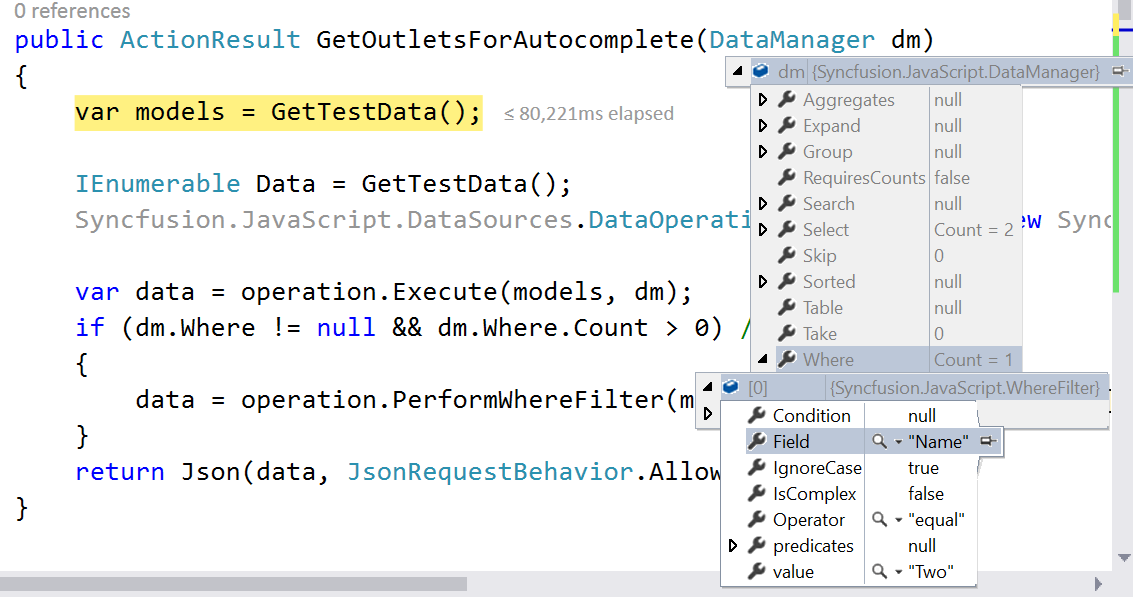Find the following output for the previous code.## How to show text on autocomplete using key value

You can set the value of autocomplete text box based on a given key value. The `SelectValueByKey` property is used to select autocomplete value based on the specified key value.

Refer to the following code sample.

``<ej:Autocomplete ID="selectState" runat="server" DataTextField="countryName" DataUniqueKeyField="index" SelectValueByKey="s2"/>``
``````namespace SyncfusionASPNETApplication1
{
public partial class _Default : Page
{
protected void Page_Load(object sender, EventArgs e)
{
this.selectState.DataSource = new LocalData().GetDataItems().ToList();
}
}
public class LocalData    {
public LocalData(string _index, string _countryName) {
this.index = _index;
this.countryName = _countryName;
}
public LocalData() { }
public string index { get; set; }
public string countryName { get; set; }

public List<LocalData> GetDataItems()
{
List<LocalData> data = new List<LocalData>();
return data;
}
}
}``````

For example, If `SelectValueByKey` property specified the value as ‘s2’, the corresponding `Text` value `Alaska` is shown in the autocomplete text.

The following output is displayed as a result of the previous code example.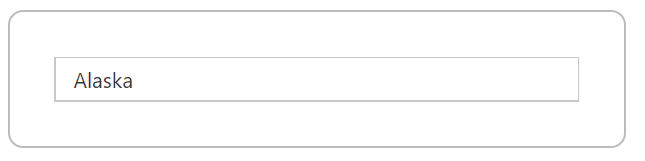If you are specifying the `SelectValueByKey` property into autocomplete control, the hidden input value holds a specified key value.

Refer to the following screenshot.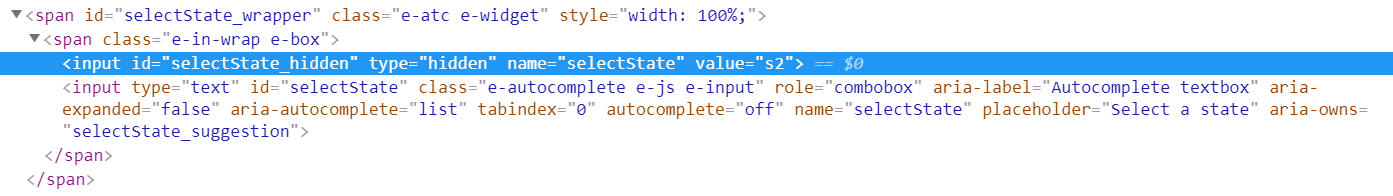### Remote data

The remote data also allows you to set the default value into autocomplete based on a given key value using the `SelectValueByKey` property.

Refer to the following code snippet.

``````<ej:Autocomplete ID="selectState" runat="server" DataTextField="countryName" DataUniqueKeyField="index" Query="ej.Query().requiresCount()" SelectValueByKey="2">
</ej:Autocomplete>``````
``````namespace SyncfusionASPNETApplication1
{
public partial class _Default : Page
{
protected void Page_Load(object sender, EventArgs e)
{
}
[WebMethod]
[ScriptMethod(ResponseFormat = ResponseFormat.Json)]
public static object Data(Syncfusion.JavaScript.DataManager value)
{
var list = new List<AutocompleteModel>();
list.Add(new AutocompleteModel() { index = "1", countryName = "One" });
list.Add(new AutocompleteModel() { index = "2", countryName = "Two" });
list.Add(new AutocompleteModel() { index = "3", countryName = "Three" });
IEnumerable Data = list;
dynamic count = list.AsQueryable().Count();
Syncfusion.JavaScript.DataSources.DataOperations operation = new Syncfusion.JavaScript.DataSources.DataOperations();
Data = operation.Execute(Data, value);
return new { result = Data, count = count };
}
}
}

namespace SyncfusionASPNETApplication1.Models
{
public class AutocompleteModel
{
[Display(Name = "index")]
public string index { get; set; }
[Display(Name = "countryName")]
public string countryName { get; set; }
}
}``````

The autocomplete `SelectValueByKey` property triggers a server-side post when using remote data on autocomplete. The server side data manger holds `where` query which contains field `name` as autocomplete `Key` property.

Find the following screenshot for the data manager where query.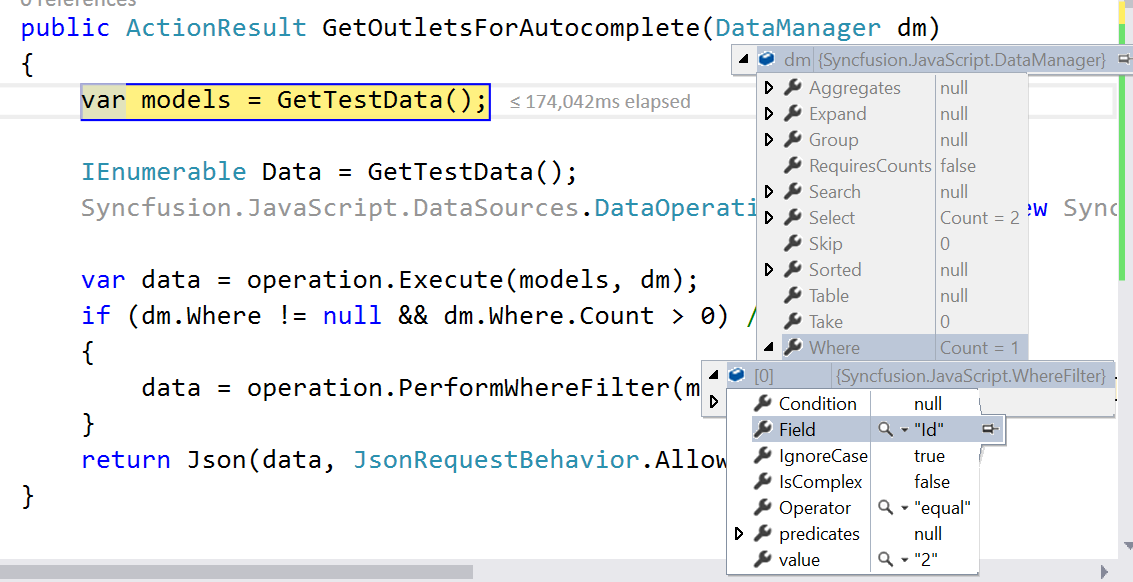Find the output for the previously given code as follows.